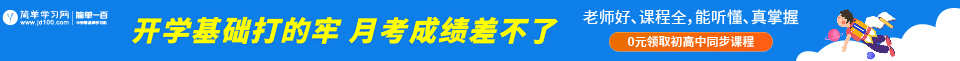# 高中三角函数公式表

来源：网络  作者：未知 今日点击：

## 第一、三角函数公式表之：半角公式## 第二、三角函数公式表之：倍角公式## 第三、三角函数公式表之：和差角公式## 第四、三角函数公式表之：积化和差公式## 第五、三角函数公式表之：和差化积公式## 第六、三角函数公式表之：诱导公式

sin(-α) = -sinα

sin(π/2-α) = cosα

sin(π/2+α) = cosα

sin(π-α) = sinα

sin(π+α) = -sinα

cos(-α) = cosα

cos(π/2-α) = sinα

cos(π/2+α) = -sinα

cos(π-α) = -cosα

cos(π+α) = -cosα

tan (—a)=-tanα

tanA= sinA/cosA

tan(π-α)=-tanα

tan(π/2+α)=-cotα

tan(π+α)=tanα

tan(π/2-α)=cotα

## 第七、高中三角函数公式表之：万能公式

sinα=2tan(α/2)/[1+tan^(α/2)]

cosα=[1-tan^(α/2)]/1+tan^(α/2)]

tanα=2tan(α/2)/[1-tan^(α/2)]

##知识拓展：三角函数公式表的灵活运用方法

（1）、了解三角函数公式的变化形式。如一下几个三角函数公式等。
（2）、三角函数恒等变形的基本策略
①常值代换：这中方法是三角函数公式在应用中常见的技巧，特别是用“1”的代换来简化三角函数公式，如1=cos2θ+sin2θ=tanx·cotx=tan45°等。
②项的分拆与角的配凑。也是三角函数公式解题比较常见的一种方法如分拆项：;
③还有一种使用三角函数公式的解题策略就是：配凑角(常用角变换)等.
④降次与升次。即三角函数中倍角公式降次与半角公式升次。
⑤化弦（切）法。将三角函数利用同角三角函数基本关系化成弦（切）。
⑥引入辅助角。三角函数会经常看到这样的公式asinθ+bcosθ=sin(θ+)，这里辅助角所在象限由a、b的符号确定，角的值由tan=确定。